A single, nonconstant force acts in the + x ‑direction on an object of mass M that is constrained to move along the x ‑axis. As

Question

A single, nonconstant force acts in the + x ‑direction on an object of mass M that is constrained to move along the x ‑axis. As a result, the object’s position as a function of time is x ( t ) = P + Q t + R t 3 How much work W is done by this force from t = 0 s to final time T ? Express the answer in terms of P , Q , R , M , and T .

in progress 0
5 months 2021-08-15T07:37:59+00:00 1 Answers 1 views 0

Velocity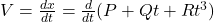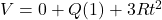V  = Q + 3Rt²

at t = 0,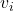= Q + 3r(0) ==> Q

at t = T,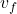= Q + 3rT²

Work done (W) = ΔKE =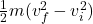W =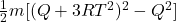W =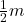[Q² + 9R²T⁴ + 2Q(3RT²) – Q²]

W =(9R²T⁴ + 6QRT²)

Explanation:

Differentiate the position.

Find the equation for speed.

Find the initial and final speed.

Use work energy theorem to find the work done by finding the change in kinetic energy.